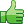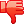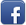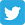# A problem with my GD class

Posted on 16th Feb 2014 07:03 pm by admin

Hello,

I created a nice (and simple for now) GD class.
The problem is , it works great on wamp, but not in my hostgator acount ( Linux )i tried it on byethost
and it didnt worked as well.

I am posting the class :

Code: <?php

/*
* Oranit 5,
* Israel.
*
*
*/

class GD
{ // start of class GD

public \$image_pointer , \$color_pointer ;

function __construct ( \$image_width, \$image_height )
{ // start of function __construct ()

\$this->image_pointer = imagecreatetruecolor (\$image_width, \$image_height);

} // end of function __construct ()

function set_color ( \$color )
{ // start of function set_color ()

\$color = substr( \$color, 1 , 6 ); // get rid of "#"
\$color = str_split( \$color , 2 ); // make 3 chanks of 2 chars.

/* Red Green Blue */
\$this->color_pointer = imagecolorallocate ( \$this->image_pointer , "0x".\$color , "0x".\$color , "0x".\$color ) ;
} // end offunction set_color ()

function fill (\$fill_x = 0 , \$fill_y = 0)
{
imagefill ( \$this->image_pointer , \$fill_x, \$fill_y , \$this->color_pointer );
}

function add_string ( \$text , \$font_type , \$location_x = 0 , \$location_y = 0 , \$method = 'h' )
{ // start of function function add_string ( )

if (\$method == 'h')
{
imagestring (\$this->image_pointer , \$font_type, \$location_x , \$location_y, \$text, \$this->color_pointer );
}
else if (\$method == 'v' )
{
imagestringup (\$this->image_pointer , \$font_type, \$location_x , \$location_y, \$text, \$this->color_pointer );
}

} // end offunction function add_string ( )

{ // start of function add_tstring ()

} // end of function add_tstring ()

// first and last

function set_line( \$f_x , \$f_y , \$l_x , \$l_y )
{ // start of function set_line()

imageline(\$this->image_pointer ,\$f_x , \$f_y , \$l_x , \$l_y , \$this->color_pointer );

} // end of function set_line()

// first and last

function rect( \$x , \$y , \$width , \$height , \$type = "fill" )
{ // start of function rect()

if ( \$type == "fill" )
{
imagefilledrectangle(\$this->image_pointer ,\$x , \$y , \$x + \$width , \$y + \$height , \$this->color_pointer );
}
else if ( \$type == "border" )
{
imagerectangle(\$this->image_pointer ,\$x , \$y , \$x + \$width , \$y + \$height , \$this->color_pointer );
}

} // end of function rect()

function create_image (\$method = 'jpeg' , \$create_file = NULL , \$quality = 100 )
{ // start of function create_image ()

if ( \$create_file != NULL )
{ // set the file name
\$create_file = \$create_file.".".\$method ;
}

if ( \$method == 'png' )
{
header ('Content-Disposition: inline; filename = "myfile.png"');
imagepng (\$this->image_pointer , \$create_file , \$quality );
}
else if ( \$method == 'jpeg' )
{
header ('Content-Disposition: inline; filename = "myfile.jpeg"');
imagejpeg (\$this->image_pointer , \$create_file , \$quality );
}

imagedestroy (\$this->image_pointer);
} // end of function create_image ()

} // end of class GD

?>

example of use:

Code: <?php
// /public_html/emp/
include ('GD.php');

\$object = new GD (400,400);
\$object->set_color ("#976686");
\$object->fill ();

\$object->set_color ("#999999");

\$object->create_image('jpeg');

?>

In wamp the picture is ok how ever on the remote servers it is black.

Thanks for the help

22645##### Other forums

PHP - HTML
Could anyone give me some GOOD sample links for php - html email tutorial.

Thanks!

Option box to change variable
Hello, i need help by making a script!

I need to write a file with option box, so a dropdown

Does design fit in FPGA ?
Hi all,

I've made a large HCC-Design. Because of the program-size the compile process with th

Remove letter from numeric textbox
I have a textbox that will search the employee database by entering in the employee ID and it will r

array help
Does anyone know how to require 10 text fields for individual grades and output class average? (10 p